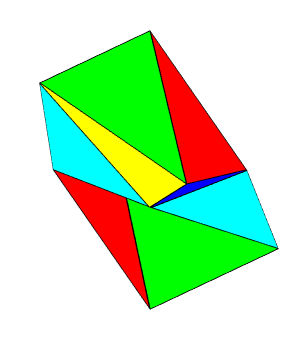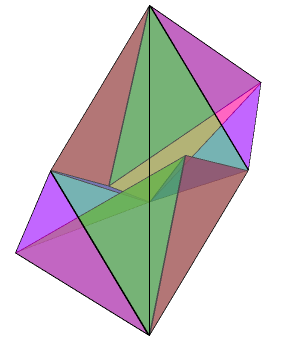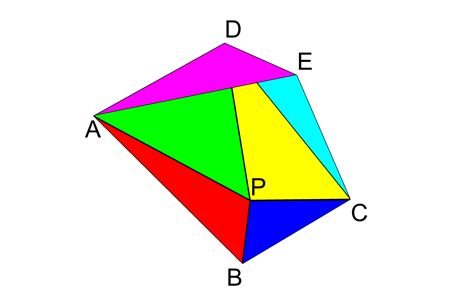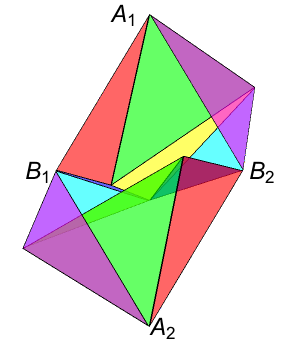# Steffen’s Polyhedron

## by Greg EganSteffen’s polyhedronchanging shape Translucent versionseen from behind

Steffen’s polyhedron is a flexible polyhedron: a polyhedron that can change shape while maintaining the shapes of all its individual faces. It has been proved that no convex polyhedron can be flexible. The first non-self-intersecting flexible polyhedron with the topology of a sphere was found by Robert Connelly in 1977, but Klaus Steffen’s version has fewer faces, with just 14 triangles, and has apparently been proved by Maksimov to be the simplest possible flexible polyhedron with triangular faces.

It’s not hard to see how Steffen’s polyhedron is constructed. First, consider the flexible shape we obtain by assembling four suitable triangles into a kind of pyramid:This shape has one degree of freedom: we can alter, for example, the length of one of the diagonals of the non-planar quadrilateral ABCD that forms the base of the pyramid. We choose AB=CD=12, BC=AD=10, AP=10, BP=5, CP=12 and DP=11. (There’s nothing special about the particular numbers 5, 10, 11 and 12, but it is crucial that opposite edges of the quadrilateral have the same lengths.)

Now, suppose we attach two more triangles to this figure, along the edges AD and CD, with the two triangles sharing an edge, DE:Specifically, we set DE=5, AE=12 and CE=10. Adding these two triangles does not introduce any more freedom to the shape, since the location of E is determined by the edges AD and CD (up to a choice of which side of the plane ACD it lies on). Perhaps surprisingly, though, even as the quadrilateral ABCD flexes, the distance BE remains constant (at 11), so the angle ECB also remains unchanged. The reason for this is that the triangle ADE is congruent to the triangle CBP, and the triangle CDE is congruent to the triangle ABP. So E is the apex of a second pyramid congruent to the first, BCE is congruent to DAP, and BE=DP=11.

To build Steffen’s polyhedron, we take two of these six-triangle figures and join them along the edges EC and CB. Specifically, we join the edge E1C1 of the first figure to the edge B2C2 of the second, and we join the edge B1C1 of the first figure to the edge E2C2 of the second. We know we can make this join regardless of the particular shapes of the two quadrilateral bases, because the angle ECB is independent of the shape. So, this assembly of 12 triangles has two degrees of freedom.Steffen’s polyhedronwith two faces removed Translucent version

To complete the polyhedron, we add triangular faces A1 B1 A2 and A2 B2 A1, and then to ensure that these faces have a constant shape, we use up one degree of freedom to impose the requirement that A1 A2 has a particular fixed length of 17. But we still have a degree of freedom left over, so the resulting polyhedron is free to flex.

(B1 B2 is fixed at 11, regardless of the specific shapes of the quadrilateral bases, but if we tried to use B1 B2 rather than A1 A2 to split A1 B1 A2 B2 into two triangular faces, they would intersect other faces of the polyhedron.)

Here’s a net for the polyhedron: a mapping of its faces into the plane, which can be printed, cut out, and folded up. Dashed lines between faces should be folded like valleys, unbroken lines like mountains. The face colours match the images above, but in order for the single-sided colours on the paper to end up on the exterior of the polyhedron, the two pyramids (viewed from the coloured side) will look like pits.Net for Steffen’s polyhedronScience Notes / Steffen’s Polyhedron / created Sunday, 23 August 2015